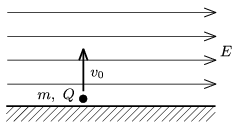Mathematical and Physical Journal
for High Schools
Issued by the MATFUND Foundation
 Already signed up? New to KöMaL?

#Problem P. 4180. (September 2009)

P. 4180. A point-like body of mass m and of charge Q is initially at rest on the horizontal tabletop as shown in the figure and is given a v0 vertical, upward speed. There is a horizontal E electric field exerted on the object. With what speed does the object hit the table when it falls back, and what is the distance between the starting point and the point where it hits the table?(4 pont)

Deadline expired on October 12, 2009.

Sorry, the solution is available only in Hungarian. Google translation

Megoldás. $\displaystyle s=\frac{2E\vert Q\vert v_{0}^{2}}{mg^2},$ $\displaystyle v=v_{0}\sqrt{1+\left(\frac{2EQ}{mg}\right)^2}.$

### Statistics:

 146 students sent a solution. 4 points: 88 students. 3 points: 33 students. 2 points: 8 students. 1 point: 6 students. 0 point: 5 students. Unfair, not evaluated: 6 solutionss.

Problems in Physics of KöMaL, September 2009